Question

# An analytical chemist is titrating 160.3mL of a 1.100M solution of acetic acid HCH3CO2 with a...

An analytical chemist is titrating 160.3mL of a 1.100M solution of acetic acid HCH3CO2 with a 0.4100M solution of NaOH. The pKa of acetic acid is 4.70. Calculate the pH of the acid solution after the chemist has added 114.1mL of the NaOH solution to it.

Note for advanced students: you may assume the final volume equals the initial volume of the solution plus the volume of NaOH solution added.

Round your answer to 2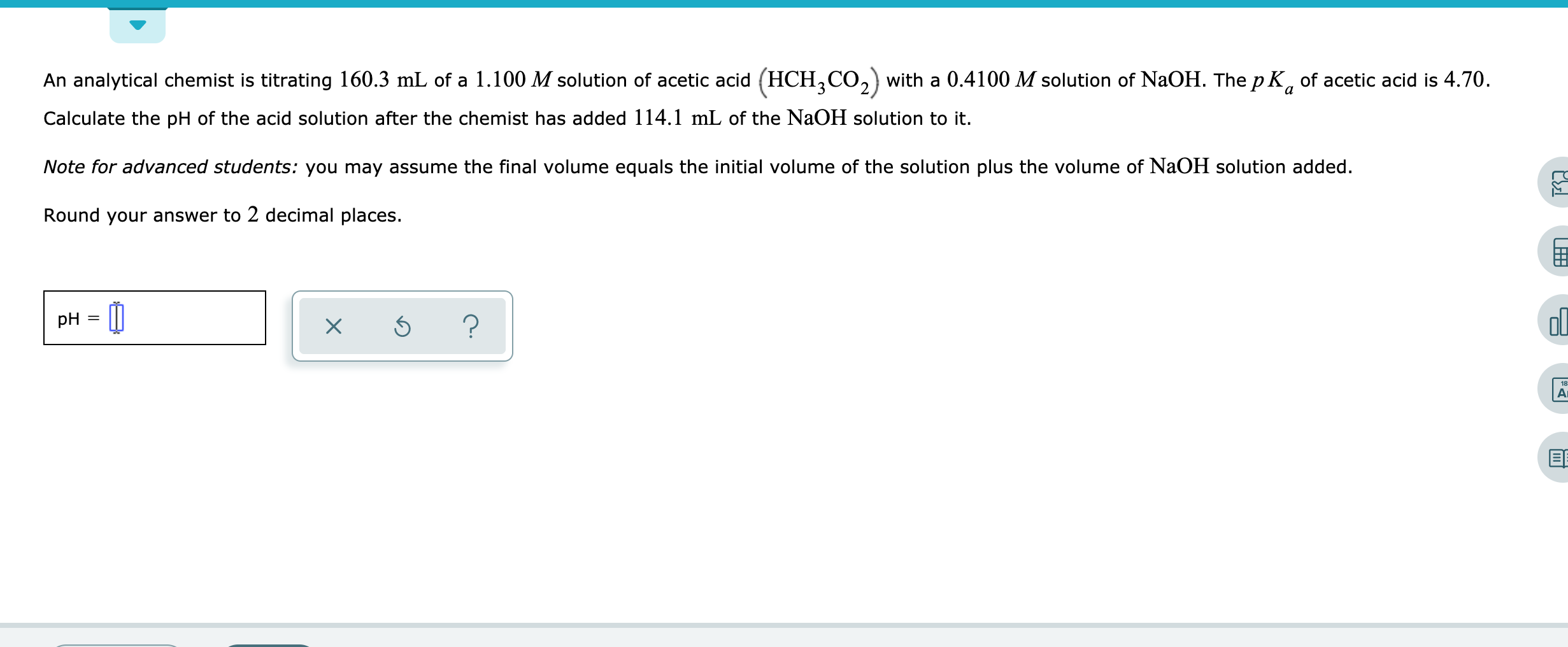decimal places.

An analytical chemist is titrating 160.3 mL of a 1.100 M solution of acetic acid (HCH2CO,) with a 0.4100 M solution of N2OH. The p K, of acetic acid is 4.70. Calculate the pH of the acid solution after the chemist has added 114.1 mL of the N2OH solution to it. Note for advanced students: you may assume the final volume equals the initial volume of the solution plus the volume of NaOH solution added Round your answer to 2 decimal places. pH X 18 Ar

Given:

M(HCH3CO2) = 1.1 M

V(HCH3CO2) = 160.3 mL

M(NaOH) = 0.41 M

V(NaOH) = 114.1 mL

mol(HCH3CO2) = M(HCH3CO2) * V(HCH3CO2)

mol(HCH3CO2) = 1.1 M * 160.3 mL = 176.33 mmol

mol(NaOH) = M(NaOH) * V(NaOH)

mol(NaOH) = 0.41 M * 114.1 mL = 46.781 mmol

We have:

mol(HCH3CO2) = 176.33 mmol

mol(NaOH) = 46.781 mmol

46.781 mmol of both will react

excess HCH3CO2 remaining = 129.549 mmol

Volume of Solution = 160.3 + 114.1 = 274.4 mL

[HCH3CO2] = 129.549 mmol/274.4 mL = 0.4721M

[CH3CO2-] = 46.781/274.4 = 0.1705M

They form acidic buffer

acid is HCH3CO2

conjugate base is CH3CO2-

use:

pH = pKa + log {[conjugate base]/[acid]}

= 4.7+ log {0.1705/0.4721}

= 4.258

#### Earn Coins

Coins can be redeemed for fabulous gifts.

Similar Homework Help Questions
• ### An analytical chemist is titrating 194.8 mL of a 0.8600 M solution of acetic acid (HCH3CO2)...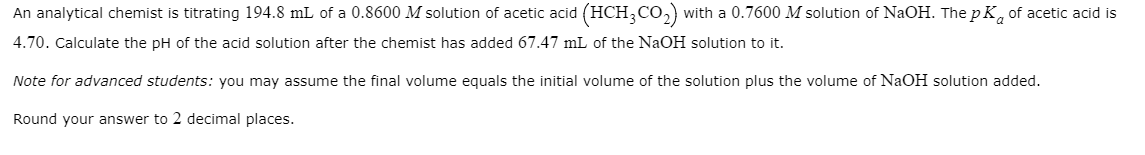An analytical chemist is titrating 194.8 mL of a 0.8600 M solution of acetic acid (HCH3CO2) with a 0.7600 M solution of NaOH. The pKof acetic acid is 4.70. Calculate the pH of the acid solution after the chemist has added 67.47 mL of the NaOH solution to it. Note for advanced students: you may assume the final volume equals the initial volume of the solution plus the volume of NaOH solution added. Round your answer to 2 decimal places.

• ### An analytical chemist is titrating 69.7 mL of a 0.3900 M solution of acetic acid (HCH,...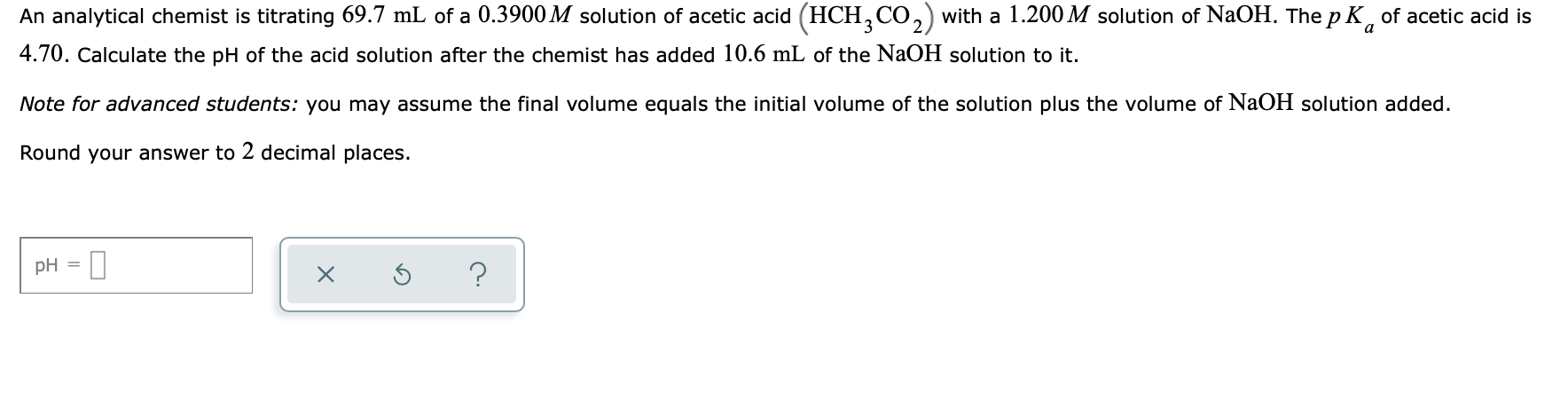An analytical chemist is titrating 69.7 mL of a 0.3900 M solution of acetic acid (HCH, CO,) with a 1.200 M solution of NaOH. The pK, of acetic acid is 4.70. Calculate the pH of the acid solution after the chemist has added 10.6 mL of the NaOH solution to it. Note for advanced students: you may assume the final volume equals the initial volume of the solution plus the volume of NaOH solution added. Round your answer to 2...

• ### An analytical chemist is titrating 106,3 ml. of a 0.9500 M solution of acetic acid (HCH,CO,)...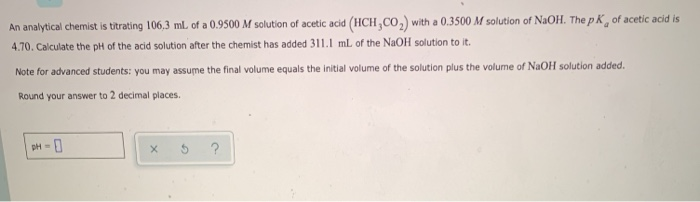An analytical chemist is titrating 106,3 ml. of a 0.9500 M solution of acetic acid (HCH,CO,) with a 0.3500 M solution of NaOH. The p K, of acetic acid is 4.70. Calculate the pH of the acid solution after the chemist has added 311.1 mL of the NaOH solution to it. Note for advanced students: you may assume the final volume equals the initial volume of the solution plus the volume of NaOH solution added. Round your answer to 2...

• ### An analytical chemist is titrating 182.1 mL. of a 0.4900 M solution of acetic acid (HCH,CO,)...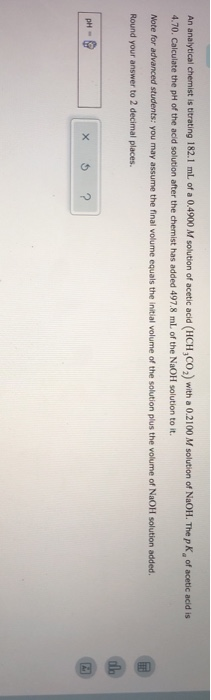An analytical chemist is titrating 182.1 mL. of a 0.4900 M solution of acetic acid (HCH,CO,) with a 0.2100 M solution of NaOH. The p K of acetic acid is 4.70. Calculate the pH of the acid solution after the chemist has added 497.8 ml of the NaOH solution to it. Note for advanced students: you may assume the final volume equals the initial volume of the solution plus the volume of NaOH solution added. Round your answer to 2...

• ### An analytical chemist is titrating 54.4 ml of a 0.9400 M solution of acetic acid (HCH,CO,)...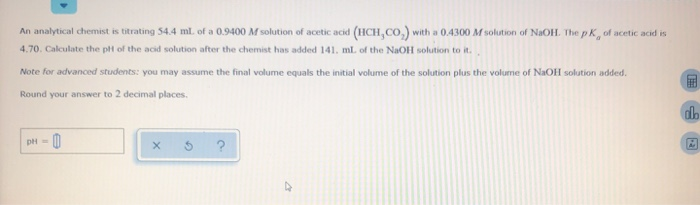An analytical chemist is titrating 54.4 ml of a 0.9400 M solution of acetic acid (HCH,CO,) with a 0.4300 M solution of NaOH. The pk of acetic acid is 4.70. Calculate the pit of the acid solution after the chemist has added 141 ml of the NaOH solution to it. Note for advanced students: you may assume the final volume equals the initial volume of the solution plus the volume of NaOH solution added. Round your answer to 2 decimal...

• ### An analytical chemist is titrating 105.5 mL of a 0.4700 M solution of acetic acid (HCH,CO)...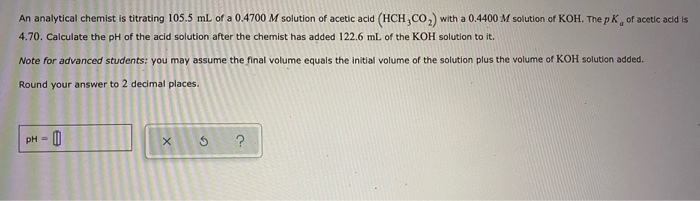An analytical chemist is titrating 105.5 mL of a 0.4700 M solution of acetic acid (HCH,CO) with a 0.4400 M solution of KOH. The pKof acetic acid is 4.70. Calculate the pH of the acid solution after the chemist has added 122.6 mL of the KOH solution to it. Note for advanced students: you may assume the final volume equals the initial volume of the solution plus the volume of KOH solution added. Round your answer to 2 decimal places....

• ### An analytical chemist is titrating 132.2 mL of a 0.9700 M solution of acetic acid (HCH,CO2)...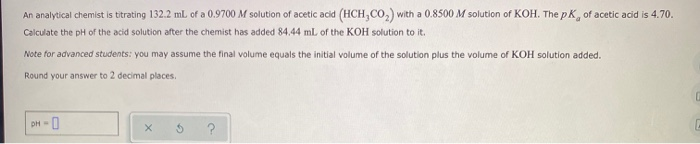An analytical chemist is titrating 132.2 mL of a 0.9700 M solution of acetic acid (HCH,CO2) with a 0.8500 M solution of KOH. The pk of acetic acid is 4.70. Calculate the pH of the acid solution after the chemist has added 84.44 mL of the KOH solution to it. Note for advanced students: you may assume the final volume equals the initial volume of the solution plus the volume of KOH solution added. Round your answer to 2 decimal...

• ### An analytical chemist is titrating 72.8 ml. of a 0.6300 M solution of acetic acid (HCH,CO,) with a 0.6600 M solutio...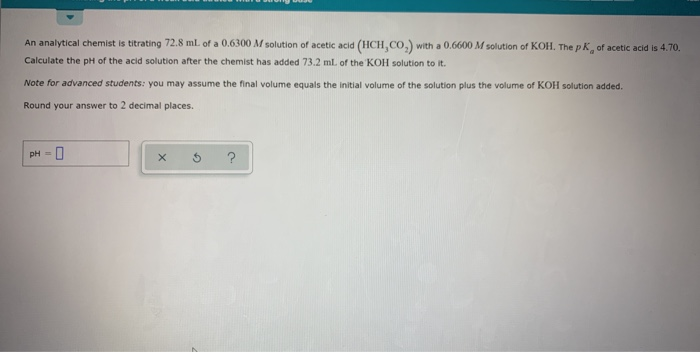An analytical chemist is titrating 72.8 ml. of a 0.6300 M solution of acetic acid (HCH,CO,) with a 0.6600 M solution of KOH. The pk, of acetic acid is 4.70. Calculate the pH of the acid solution after the chemist has added 73.2 ml of the KOH solution to it Note for advanced students: you may assume the final volume equals the initial volume of the solution plus the volume of KOH solution added. Round your answer to 2 decimal...

• ### An analytical chemist is titrating 171.9 mL of a 1.100 M solution of cyanic acid (HCNO)...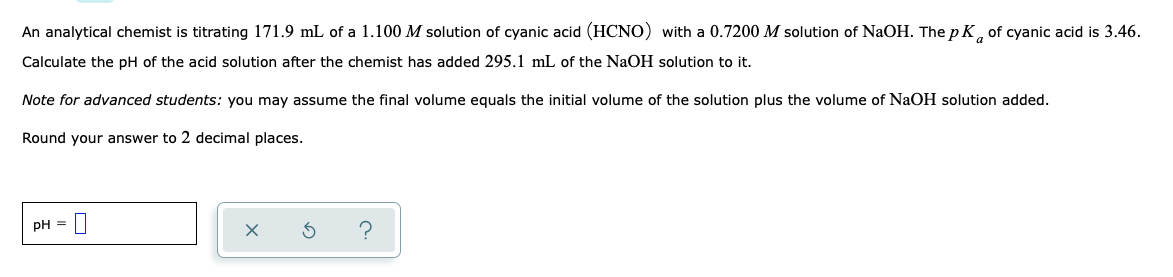An analytical chemist is titrating 171.9 mL of a 1.100 M solution of cyanic acid (HCNO) with a 0.7200 M solution of NaOH. The pK, of cyanic acid is 3.46. Calculate the pH of the acid solution after the chemist has added 295.1 mL of the NaOH solution to it. Note for advanced students: you may assume the final volume equals the initial volume of the solution plus the volume of NaOH solution added. Round your answer to 2 decimal...

• ### An analytical chemist is titrating 195.2mL of a 0.8100M solution of benzoic acid HC6H5CO2 with a...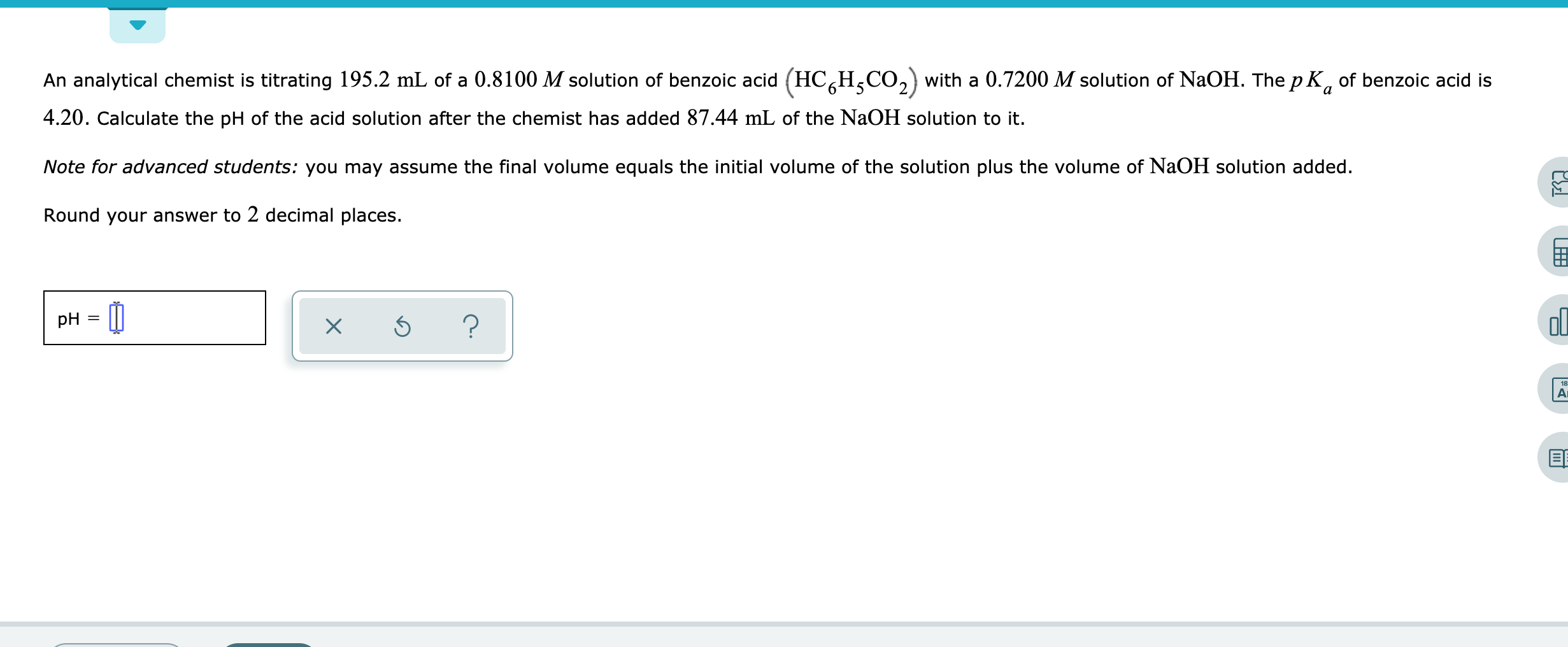An analytical chemist is titrating 195.2mL of a 0.8100M solution of benzoic acid HC6H5CO2 with a 0.7200M solution of NaOH. The pKa of benzoic acid is 4.20. Calculate the pH of the acid solution after the chemist has added 87.44mL of the NaOH solution to it. Note for advanced students: you may assume the final volume equals the initial volume of the solution plus the volume of NaOH solution added. Round your answer to 2 decimal places. An analytical chemist...